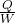## An air conditioner is a refrigerator with the inside of the house acting as the cold reservoir and the outside atmosphere acting as the hot

Question

An air conditioner is a refrigerator with the inside of the house acting as the cold reservoir and the outside atmosphere acting as the hot reservoir. Assume that an air conditioner consumes 1.25 * 103 W of electrical power and that it can be idealized as a reversible Carnot refrigerator. If the coefficient of performance of this device is 1.75, how much heat can be extracted from the house in a day

in progress 0
6 months 2021-08-09T10:51:53+00:00 1 Answers 22 views 0

1.89*10^8 J

Explanation:

The coefficient of performance of this device iswhere Q .is the useful heat supplied or removed by the considered system and W  is the work required by the considered system.

Step 1

Coeffiecient of perfromance for cooling (COPC) = 1.75 =.

Q = 1.75W

Step 2

We convert day into seconds:

1 day = 24 hrs = 86400 seconds

Step 3

Heat that can be extracted from the house in a day is:

Q = 1.75 * 1.25 * 10^3 * 86400 =  189000000 = 1.89*10^8 J